SSC CGL Previous Year Questions: Algebra- 4

# SSC CGL Previous Year Questions: Algebra- 4 - SSC CGL

Test Description

## 40 Questions MCQ Test SSC CGL (Tier - 1) - Previous Year Papers (Topic Wise) - SSC CGL Previous Year Questions: Algebra- 4

SSC CGL Previous Year Questions: Algebra- 4 for SSC CGL 2023 is part of SSC CGL (Tier - 1) - Previous Year Papers (Topic Wise) preparation. The SSC CGL Previous Year Questions: Algebra- 4 questions and answers have been prepared according to the SSC CGL exam syllabus.The SSC CGL Previous Year Questions: Algebra- 4 MCQs are made for SSC CGL 2023 Exam. Find important definitions, questions, notes, meanings, examples, exercises, MCQs and online tests for SSC CGL Previous Year Questions: Algebra- 4 below.
Solutions of SSC CGL Previous Year Questions: Algebra- 4 questions in English are available as part of our SSC CGL (Tier - 1) - Previous Year Papers (Topic Wise) for SSC CGL & SSC CGL Previous Year Questions: Algebra- 4 solutions in Hindi for SSC CGL (Tier - 1) - Previous Year Papers (Topic Wise) course. Download more important topics, notes, lectures and mock test series for SSC CGL Exam by signing up for free. Attempt SSC CGL Previous Year Questions: Algebra- 4 | 40 questions in 40 minutes | Mock test for SSC CGL preparation | Free important questions MCQ to study SSC CGL (Tier - 1) - Previous Year Papers (Topic Wise) for SSC CGL Exam | Download free PDF with solutions
 1 Crore+ students have signed up on EduRev. Have you?
SSC CGL Previous Year Questions: Algebra- 4 - Question 1

### If (4x/3) + 2P = 12 for what value of P, x = 6 ?        (SSC CGL 1st Sit. 2013)

Detailed Solution for SSC CGL Previous Year Questions: Algebra- 4 - Question 1

When x = 6.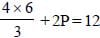⇒ 8 + 2P = 12
⇒ 2P = 12 – 8 = 4 Þ P = 2

SSC CGL Previous Year Questions: Algebra- 4 - Question 2

### If (x – 3)2 + (y – 5)2 + (z – 4)2 = 0, then the value of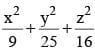is        (SSC CGL 1st Sit. 2013)

Detailed Solution for SSC CGL Previous Year Questions: Algebra- 4 - Question 2

(x – 3)2 + (y – 5)2 + (z – 42)= 0
⇒ x – 3 = 0 ⇒ x = 3
y – 5 = 0 ⇒ y = 5
z – 4 = 0 ⇒ z = 4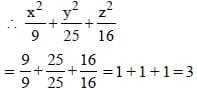SSC CGL Previous Year Questions: Algebra- 4 - Question 3

### If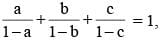then the value of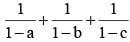is         (SSC CGL 1st Sit. 2013)

Detailed Solution for SSC CGL Previous Year Questions: Algebra- 4 - Question 3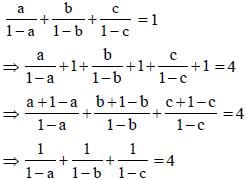SSC CGL Previous Year Questions: Algebra- 4 - Question 4

The total cost of 8 buckets and 5 mugs is ₹ 92 and the total cost of 5 buckets and 8 mugs is  ₹ 77. Find the cost of 2 mugs and 3 buckets.      (SSC CGL 1st Sit. 2013)

Detailed Solution for SSC CGL Previous Year Questions: Algebra- 4 - Question 4

C.P. of 1 bucket = ₹ x
C.P. of 1 mug = ₹ y
∴ 8x + 5y = 92 ...(i)
5x + 8y = 77 ...(ii)
By equation (i) × 5 – equation (ii) × 8,
40x + 25y – 40x – 64y = 460 – 616
⇒ –39y = –156
⇒ y = 4
From equation (i),
8x + 20 = 92
⇒ 8x = 92 – 20 = 72
⇒ x = 9
∴ C.P. of 2 mugs and 3 buckets
= 2 × 4 + 3 × 9
= 8 + 27 = ₹ 35

SSC CGL Previous Year Questions: Algebra- 4 - Question 5

The sum of the ages of Puneet and his father is 45 years and the product of their ages is 126. What is the age of Puneet?             (SSC CGL1st Sit. 2013)

Detailed Solution for SSC CGL Previous Year Questions: Algebra- 4 - Question 5

Let Puneet’s age = x yr.
Let Puneet’s father age = y yr.
x + y = 45 ⇒ y = (45 – x)
According to question,
xy = 126
Putting the value of y.
(x) (45 – x) = 126
45x – x2 = 126
x2 – 45x + 126 = 0
x2 – 42x – 3x + 126 = 0
x (x – 42) – 3 (x – 42) = 0
x = 3, x = 42
Hence, Puneet’s age is 3yr.

SSC CGL Previous Year Questions: Algebra- 4 - Question 6

If a = 0, b ≠ 0, c ≠ 0, then the equation ax + by + c = 0 represents a line parallel to        (SSC CGL1st Sit. 2013)

Detailed Solution for SSC CGL Previous Year Questions: Algebra- 4 - Question 6

It a = 0, b ≠ 0, c ≠ 0, then equation ax + by + c = 0 represents a line parallel to x-axis.

SSC CGL Previous Year Questions: Algebra- 4 - Question 7

If ‘a’ be a positive number, then the least value of a + (1/a) is      (SSC CGL 1st Sit. 2013)

Detailed Solution for SSC CGL Previous Year Questions: Algebra- 4 - Question 7

The least value of a + (1/a) is 2 where a = 1.

SSC CGL Previous Year Questions: Algebra- 4 - Question 8

If x = 3 + 2√2, then the value of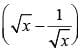is       (SSC CGL1st Sit. 2013)

Detailed Solution for SSC CGL Previous Year Questions: Algebra- 4 - Question 8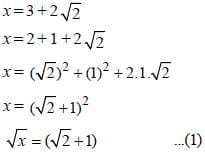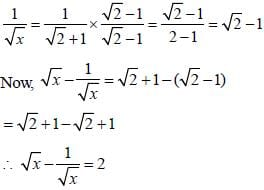SSC CGL Previous Year Questions: Algebra- 4 - Question 9

If x + (4/x) = 4, find the value of x3 + (4/x3).        (SSC CGL 1st Sit. 2013)

Detailed Solution for SSC CGL Previous Year Questions: Algebra- 4 - Question 9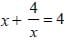x2 + 4 = 4x ⇒ x2 – 4x + 4 = 0 ⇒ (x – 2)2 = 0
x = 2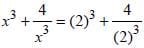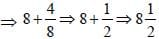SSC CGL Previous Year Questions: Algebra- 4 - Question 10

If a + b + c = 0, find the value of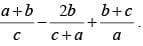(SSC CGL 1st Sit. 2013)

Detailed Solution for SSC CGL Previous Year Questions: Algebra- 4 - Question 10

a + b + c = 0
i.e. a = – (b + c); b = – (c + a); c = – (a + b)
Now,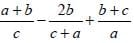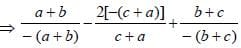⇒ – 1 + 2 – 1 = 0

SSC CGL Previous Year Questions: Algebra- 4 - Question 11

If 3x – 1/4y = 6, then the value of 4x – 1/3y is        (SSC CGL 1st Sit. 2013)

Detailed Solution for SSC CGL Previous Year Questions: Algebra- 4 - Question 11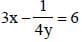12 xy – 1 = 24y
Now,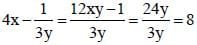SSC CGL Previous Year Questions: Algebra- 4 - Question 12

The expression x4 – 2x2 + k will be a perfect square when the value of k is       (SSC CGL1st Sit. 2013)

Detailed Solution for SSC CGL Previous Year Questions: Algebra- 4 - Question 12

x4 – 2x2 + k
(x2)2 – 2x2 + k ⇒ (x2)2 – 2.1. x2 + k
For above expression to make a perfect square, the k value is equal to 1.

SSC CGL Previous Year Questions: Algebra- 4 - Question 13

An equation of the form ax + by + c = 0 where a ≠ 0, b ≠ 0, c = 0 represents a straight line which passes through      (SSC CGL1st Sit. 2013)

Detailed Solution for SSC CGL Previous Year Questions: Algebra- 4 - Question 13

ax + by +c = 0
When c = 0,
ax + by = 0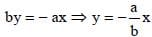When x = 0, y = 0 i,e. this line passes through the origin (0, 0).

SSC CGL Previous Year Questions: Algebra- 4 - Question 14

If a2 + b2 + 4c2 = 2(a + b – 2c) – 3 and a, b, c are real, then the value of (a2 + b2 + c2) is       (SSC CGL 1st Sit. 2013)

Detailed Solution for SSC CGL Previous Year Questions: Algebra- 4 - Question 14

a2 + b2 + 4c2 = 2a +2b – 4c – 3
⇒ a2 + b2 + 4c2 – 2a –2b + 4c + 3 = 0
⇒ a2 – 2a + 1 + b2 – 2b + 1 + 4c2 + 4c + 1 = 0
⇒ (a –1)2 + (b – 1)2 + (2c + 1)2 = 0
∴ a – 1 = 0 ⇒ a = 1;
b – 1 = 0 ⇒ b = 1;
2c + 1 = 0 ⇒ c = -(1/2)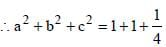= 2(1/4)

SSC CGL Previous Year Questions: Algebra- 4 - Question 15

If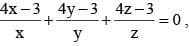then the value of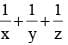is      (SSC CGL 1st Sit. 2013)

Detailed Solution for SSC CGL Previous Year Questions: Algebra- 4 - Question 15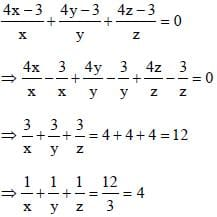SSC CGL Previous Year Questions: Algebra- 4 - Question 16

If x2 – 3x + 1 = 0, then the value of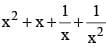is       (SSC CGL 1st Sit. 2013)

Detailed Solution for SSC CGL Previous Year Questions: Algebra- 4 - Question 16

x2 – 3x + 1 = 0
⇒ x2 + 1 = 3x
Dividing both sides by x,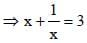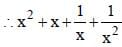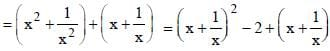= 9 – 2 + 3 = 10

SSC CGL Previous Year Questions: Algebra- 4 - Question 17

The third proportional to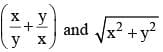is     (SSC CGL 2nd Sit. 2013)

Detailed Solution for SSC CGL Previous Year Questions: Algebra- 4 - Question 17

Third proportional of a and b
a : b : c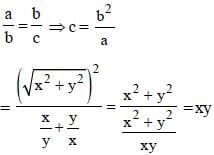SSC CGL Previous Year Questions: Algebra- 4 - Question 18

If x + y + z = 6 and x2 + y2 + z2 = 20 then the value of x3 + y3 + z3 – 3xyz is        (SSC CGL 2nd Sit. 2013)

Detailed Solution for SSC CGL Previous Year Questions: Algebra- 4 - Question 18

x + y + z = 6
On squaring,
x2 + y2 + z2 + 2xy + 2zy + 2zx = 36
⇒ 20 + 2 (xy + yz + zx) = 36
⇒ xy + yz + zx = 8
∴ x3 + y3 + z3 – 3xyz
= (x + y + z) (x2 + y2 + z2 – xy – yz – zx)
= 6 (20 – 8) = 72

SSC CGL Previous Year Questions: Algebra- 4 - Question 19

The minimum value of  (x – 2) (x – 9) is        (SSC CGL 2nd Sit. 2013)

Detailed Solution for SSC CGL Previous Year Questions: Algebra- 4 - Question 19

Expression = (x – 2) (x – 9)
= x2 – 11x + 18 = ax2 + bx + c
Minimum value =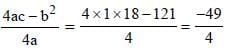SSC CGL Previous Year Questions: Algebra- 4 - Question 20

If a3 – b3 – c3 = 0 then the value of a9 – b9 – c9 – 3a3b3c3 is       (SSC CGL 2nd Sit. 2013)

Detailed Solution for SSC CGL Previous Year Questions: Algebra- 4 - Question 20

If a + b + c = 0, then
a3 + b3 + c3 – 3abc = 0

SSC CGL Previous Year Questions: Algebra- 4 - Question 21

If xy (x + y) = 1 then, the value of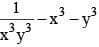is       (SSC CGL 2nd Sit. 2013)

Detailed Solution for SSC CGL Previous Year Questions: Algebra- 4 - Question 21

xy (x + y) = 1
⇒ x + y = 1/xy
Cubing both sides,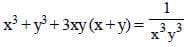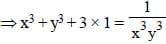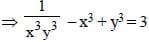SSC CGL Previous Year Questions: Algebra- 4 - Question 22

If a + b + c = 2s, then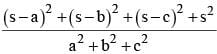is equal to       (SSC CGL 2nd Sit. 2013)

Detailed Solution for SSC CGL Previous Year Questions: Algebra- 4 - Question 22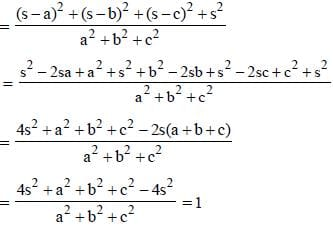SSC CGL Previous Year Questions: Algebra- 4 - Question 23

If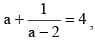then the value of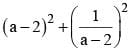is        (SSC CGL 2nd Sit. 2013)

Detailed Solution for SSC CGL Previous Year Questions: Algebra- 4 - Question 23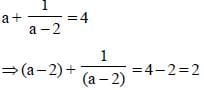On squaring,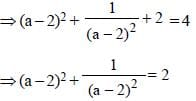SSC CGL Previous Year Questions: Algebra- 4 - Question 24

If the number p is 5 more than q and the sum of the squares of p and q is 55, then the product of p and q is       (SSC CGL 2nd Sit. 2013)

Detailed Solution for SSC CGL Previous Year Questions: Algebra- 4 - Question 24

p = q + 5
⇒ p – q = 5
p2 + q2 = 55
∴ (p – q)2 + 2pq = 55
⇒ 25 + 2 pq = 55
⇒ 2 pq = 30
⇒ pq = 15

SSC CGL Previous Year Questions: Algebra- 4 - Question 25

If (x + 7954 × 7956) be a square number, then the value of ‘x’ is        (SSC CGL 2nd Sit. 2013)

Detailed Solution for SSC CGL Previous Year Questions: Algebra- 4 - Question 25

x + 7954 × 7956
x + 7954 (7954 + 2)
x + 79542 + 2 × 7954 × 1
Putting x = 1
(x + 7954)2 or (1 + 7954)2

SSC CGL Previous Year Questions: Algebra- 4 - Question 26

Equation of the straight line parallel to x-axis and also 3 units below x-axis is :        (SSC CGL 1st Sit. 2013)

Detailed Solution for SSC CGL Previous Year Questions: Algebra- 4 - Question 26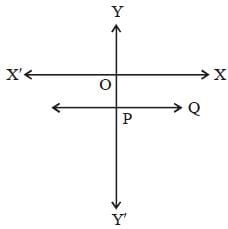∴ Equation is : y = –3

SSC CGL Previous Year Questions: Algebra- 4 - Question 27

If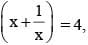then the value of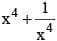is :        (SSC CGL 1st Sit. 2013)

Detailed Solution for SSC CGL Previous Year Questions: Algebra- 4 - Question 27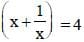On squaring both sides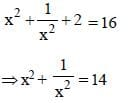On squaring again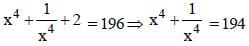SSC CGL Previous Year Questions: Algebra- 4 - Question 28

If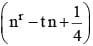be a perfect square, then the values of t are:       (SSC CGL 1st Sit. 2013)

Detailed Solution for SSC CGL Previous Year Questions: Algebra- 4 - Question 28

For nr – tn + (1/4)to be a perfect square, r = 2 and t = ± 1
Look :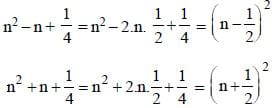SSC CGL Previous Year Questions: Algebra- 4 - Question 29

If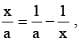then the value of x – x2 is :      (SSC CGL 1st Sit. 2013)

Detailed Solution for SSC CGL Previous Year Questions: Algebra- 4 - Question 29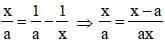⇒ x2 = x – a ⇒ x – x2 = a

SSC CGL Previous Year Questions: Algebra- 4 - Question 30

If a2 + b2 + c2 + 3 = (2 (a – b – c), then the value of 2a – b + c is :        (SSC CGL 1st Sit. 2013)

Detailed Solution for SSC CGL Previous Year Questions: Algebra- 4 - Question 30

a2 + b2 + c2 + 3
= 2a – 2b – 2c
⇒ a2 – 2a + 1 + b2 + 2b + 1 + c2 * + 2c + 1 = 0
⇒ (a – 1)2 + (b + 1)2 + (c + 1)2 = 0
∴ a – 1 = 0 ⇒ a = 1
b + 1 = 0 ⇒ b = –1
c + 1 = 0 ⇒ c = –1
∴ 2a – b + c = 2 + 1 – 1 = 2

SSC CGL Previous Year Questions: Algebra- 4 - Question 31

If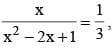then the value of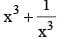is:         (SSC CGL 1st Sit. 2013)

Detailed Solution for SSC CGL Previous Year Questions: Algebra- 4 - Question 31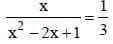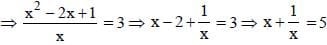On cubing both sides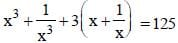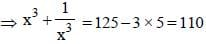SSC CGL Previous Year Questions: Algebra- 4 - Question 32

If p – 2q = 4, then the value of p3 – 8q3 – 24pq – 64 is :       (SSC CGL 1st Sit. 2013)

Detailed Solution for SSC CGL Previous Year Questions: Algebra- 4 - Question 32

p – 21 = 4
cubing both sides,
( p – 2q)3 = 64
⇒ p3 – 8q3 + 3p. 4q2 – 3p2 . 2q = 64
⇒ p3 – 8q3 + 12pq2 – 6p2q = 64
⇒ p3 – 8q3 – 6pq (p – 2q) = 64
⇒ p3 – 8q3 – 6pq × 4 = 64
⇒ p3 – 8q3 – 24pq – 64 = 0

SSC CGL Previous Year Questions: Algebra- 4 - Question 33

A man buys 3 cows and 18 goats in ₹ 47,200. Instead if he would have bought 8 cows and 3 goats, he had to pay ₹ 53,000 mroe. Cost of one cow is :       (SSC CGL 1st Sit. 2013)

Detailed Solution for SSC CGL Previous Year Questions: Algebra- 4 - Question 33

C.P of 1 cow = ₹ x
C.P of a goat = ₹ y
3x + 8y = 47200 ...(i)
⇒ 8x + 3y = 100200 ...(ii)
By equation (i) × 3 – (ii) × 8, 9x + 24y – 64x – 24y
= 141600 – 801600
⇒ 55x = 660000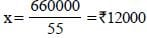SSC CGL Previous Year Questions: Algebra- 4 - Question 34

If a + b + c = 9 (where a, b, c are real numbers), then the minimum value of       (SSC CHSL 2013)
a2 + b2 + c2 is

Detailed Solution for SSC CGL Previous Year Questions: Algebra- 4 - Question 34

a2 +b2 + c2 = (a + b + c)2 - 2(ab +bc + ca)
= 92 - 2(ab + bc + ca)
a2 + b2 + c2 will be minimum if ab + bc + ca is maximum.
ab + bc + ca is maximum when a = 3, b = 3, and c = 3.
[∵ a + b + c = 9]
∴ minimum value of a2 + b2 + c2
= 81 – 2(3 × 3 + 3 × 3 + 3 × 3)
= 81 – 54 = 27

SSC CGL Previous Year Questions: Algebra- 4 - Question 35

The equation cos2θ =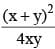is only possible when       (SSC CHSL 2013)

Detailed Solution for SSC CGL Previous Year Questions: Algebra- 4 - Question 35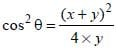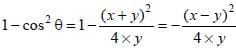sin2θ cannot be – ve
Both sin2θ and cos2θ will be +ve when x = y

SSC CGL Previous Year Questions: Algebra- 4 - Question 36

In a two-digit number, the digit at the unit's place is 1 less than twice the digit at the ten’s place. If the digits at unit’s and ten’s place are interchanged, the difference between the new and the original number is less than the original number by 20. The original number is       (SSC CHSL 2013)

Detailed Solution for SSC CGL Previous Year Questions: Algebra- 4 - Question 36

Since two digit number = 10x + y
According to question → y = 2x – 1 ..(i)
When digits are interchanged then new number
= 10y + x
then original number – [new number – original number]
= 20
→ 10x + y – [10y + x – (10x + y)] = 20
→ 10x + y – 10y – x + 10x + y = 20
19x – 8y = 20
19x – 8(2x – 1) = 20 (Using eq. (i))
19x – 16x + 8 = 20
3x = 12 → x = 4
From (i) y = 2 × 4 – 1 = y = 7
∴ original number = 10x + y = 10×4 + 7 = 47

SSC CGL Previous Year Questions: Algebra- 4 - Question 37

Divide 50 into two parts so that the sum of their reciprocals is 1/12.        (SSC CHSL 2013)

Detailed Solution for SSC CGL Previous Year Questions: Algebra- 4 - Question 37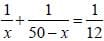x2 – 50x + 600 = 0
x2 – 30x – 20x + 600 = 0
x(x – 30) – 20(x – 30) = 0
x = 30, 20

SSC CGL Previous Year Questions: Algebra- 4 - Question 38

If a2 + b2 = 5ab, then the value of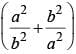is :      (SSC Sub. Ins. 2013)

Detailed Solution for SSC CGL Previous Year Questions: Algebra- 4 - Question 38

a2 + b2 = 5ab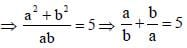On squaring both sides.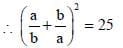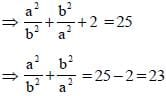SSC CGL Previous Year Questions: Algebra- 4 - Question 39

If the square of the sum of two numbers is equal to 4 times of their product. then the ratio of these numbers is :         (SSC Sub. Ins. 2013)

Detailed Solution for SSC CGL Previous Year Questions: Algebra- 4 - Question 39

Let the number be x and y.
According to question,
(x + y)2 = 4xy
⇒ x2 + y2 + 2xy – 4xy = 0
⇒ (x – y)2 = 0
⇒ x = y

SSC CGL Previous Year Questions: Algebra- 4 - Question 40

One of the factors of the expression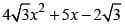is:       (SSC Sub. Ins. 2013)

Detailed Solution for SSC CGL Previous Year Questions: Algebra- 4 - Question 40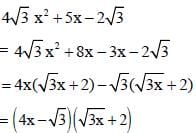## SSC CGL (Tier - 1) - Previous Year Papers (Topic Wise)

250 tests
Information about SSC CGL Previous Year Questions: Algebra- 4 Page
In this test you can find the Exam questions for SSC CGL Previous Year Questions: Algebra- 4 solved & explained in the simplest way possible. Besides giving Questions and answers for SSC CGL Previous Year Questions: Algebra- 4, EduRev gives you an ample number of Online tests for practice

250 tests